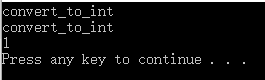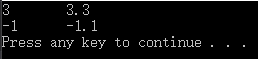# 1、operator作类的转换函数

my_class a = my_class(int i);

• 转换函数必须是类方法
• 转换函数不能指定返回类型
• 转换函数不能有参数

下面是一个类转化函数的例子

#include <iostream>

using namespace std;

class my_class
{
public:
operator int()//定义了一个将类转化为int的转换函数
{
cout << "convert_to_int" << endl;
return 1;
}
};

int main()
{
my_class a;
int i_a = (int)a;//第一次显式的转换
cout << a << endl;//第二次隐式的转换

return 0;
}#include <iostream>

using namespace std;

class my_class
{
public:
operator double()//定义了一个将类转化为double的转换函数
{
cout << "convert_to_double" << endl;
return 1.1;
}

operator int()//定义了一个将类转化为int的转换函数
{
cout << "convert_to_int" << endl;
return 1;
}
};

int main()
{
my_class a;
cout << a << endl;//报错

return 0;
}

有多个运算符 "<<" 与这些操作数匹配

cout << (double)a << endl;

explicit operator double()
{
cout << "convert_to_double" << endl;
return 1.1;
}

# 2、operator在类中重载运算符

• 类型转换：operator <类型>()
• 运算符重载：<类型> operator <运算符>(<参数表>)

#include <iostream>

using namespace std;

class MyClass
{
public:
MyClass() {}
MyClass(int a, double b):a_(a),b_(b){}
~MyClass(){}

int get_a() { return a_; }
double get_b() { return b_; }

{
MyClass sum;
return sum;
}

friend MyClass operator-(const MyClass &A,const MyClass &B)//以友元方式重载-
{
MyClass diff;
diff.a_ = A.a_ - B.a_;
diff.b_ = A.b_ - B.b_;
return diff;
}

private:
int a_;
double b_;
};

int main()
{
MyClass A(1, 1.1);
MyClass B(2, 2.2);
MyClass sum = A + B;
MyClass diff = A - B;
cout << sum.get_a() << "\t" << sum.get_b() << endl;
cout << diff.get_a() << "\t" << diff.get_b() << endl;

return 0;
}06-118万+
05-294万+06-115万+
03-226724
07-061720
09-123580
09-245918